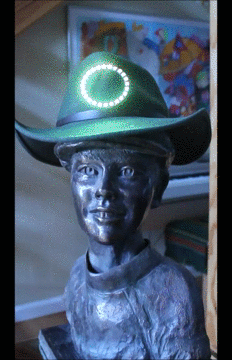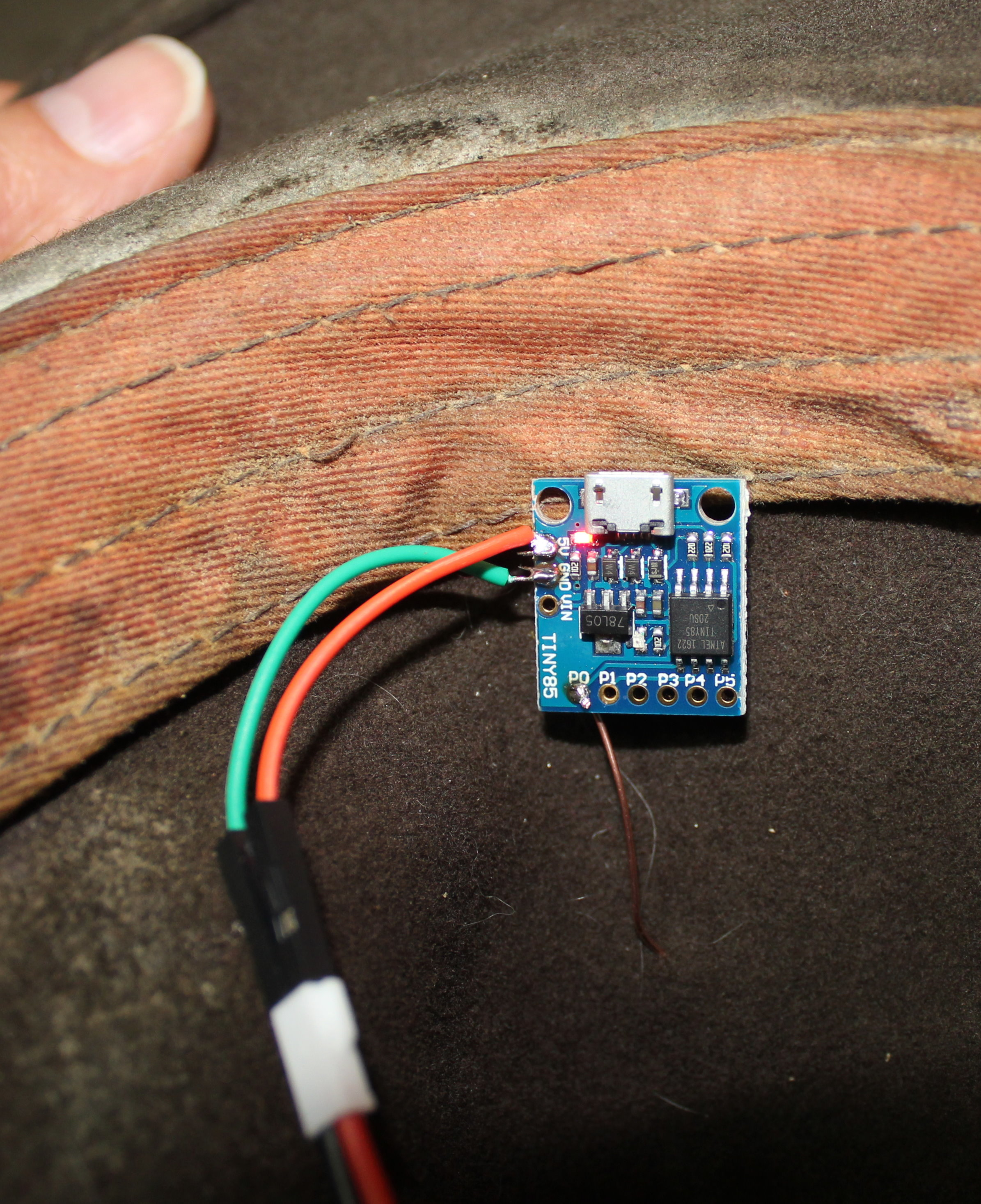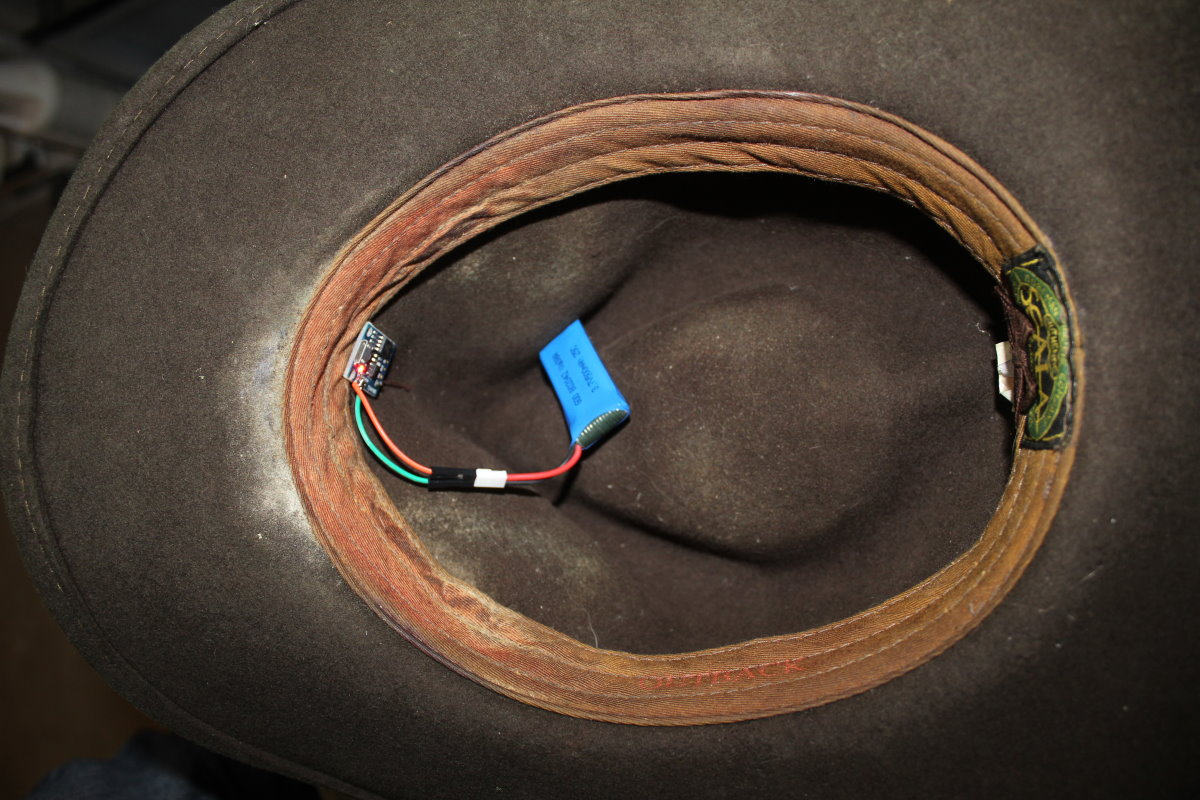# Orbiting Comet HatThis project puts a circle of 24 RGB LEDs on a hat, and makes them rotate and gradually dim, resulting in what we call a Comet pattern.

Like most NeoPixel projects, the construction is very simple, with only a few connections to solder. All of the tricky stuff happens in the software.

We don't need a lot of processing power, so one of the smaller (cheaper) Arduino boards will do just fine, such as the Pro Mini we used in some previous projects. In this case, however, I chose the Digispark ATTiny85, which is a little more complicated to program, as it involves a bit of fussing around with the IDE to set up the programming environment. This is covered well in the Digispark documentation, but beginners are better off using the Arduino Nano, or the Arduino Pro Mini.

The ring of LEDs has three inputs: power, ground, and data in. We solder three bare copper wires to these holes, so the wires stick out straight from the back of the circle. We will poke three holes in the hat with a large needle, and push the wires through the felt, so the circle lies flat against the front of the hat.Inside the hat, the bare copper wires are soldered to the 5 volt, Ground, and P0 holes of the ATTiny85. Two more wires are soldered to the 5 volt and Ground holes so we can connect the battery.The computer and the battery both tuck into the sweatband of the hat, so it can be worn comfortably, without the wearer even feeling them. We use a small 500 milliampere-hour rechargeable lithium polymer battery.

The code is very similar to our previous NeoPixel projects. It includes the NeoPixel library, defines the HSV to RGB color conversion routine, and has a tiny bit of code in the loop() routine that sets the color and brightness of the LEDs each time it is called. The entire code looks like this:

enum { PIN = 0, NUMPIXELS = 24, FULLY_SATURATED = 255 };
int delayval = 4;

typedef struct RgbColor
{
unsigned char r;
unsigned char g;
unsigned char b;
} RgbColor;

typedef struct HsvColor
{
unsigned char h;
unsigned char s;
unsigned char v;
HsvColor( void ) { h = s = v = 0; }
HsvColor( int a, int b, int c )
{
h = a;
s = b;
v = c;
}
} HsvColor;

RgbColor HsvToRgb( HsvColor hsv )
{
RgbColor rgb;
unsigned char region, p, q, t;
unsigned int h, s, v, remainder;

if( hsv.s == 0 )
{
rgb.r = hsv.v;
rgb.g = hsv.v;
rgb.b = hsv.v;
return rgb;
}

// converting to 16 bit to prevent overflow
h = hsv.h;
s = hsv.s;
v = hsv.v;

region = h / 43;
remainder = (h - (region * 43)) * 6;

p = (v * (255 - s)) >> 8;
q = (v * (255 - ((s * remainder) >> 8))) >> 8;
t = (v * (255 - ((s * (255 - remainder)) >> 8))) >> 8;

switch (region)
{
case 0:
rgb.r = v;
rgb.g = t;
rgb.b = p;
break;
case 1:
rgb.r = q;
rgb.g = v;
rgb.b = p;
break;
case 2:
rgb.r = p;
rgb.g = v;
rgb.b = t;
break;
case 3:
rgb.r = p;
rgb.g = q;
rgb.b = v;
break;
case 4:
rgb.r = t;
rgb.g = p;
rgb.b = v;
break;
default:
rgb.r = v;
rgb.g = p;
rgb.b = q;
break;
}

return rgb;
}

HsvColor RgbToHsv( RgbColor rgb )
{
HsvColor hsv;
unsigned char rgbMin, rgbMax;

rgbMin = rgb.r < rgb.g ? (rgb.r < rgb.b ? rgb.r : rgb.b) : (rgb.g < rgb.b ? rgb.g : rgb.b);
rgbMax = rgb.r > rgb.g ? (rgb.r > rgb.b ? rgb.r : rgb.b) : (rgb.g > rgb.b ? rgb.g : rgb.b);

hsv.v = rgbMax;
if( hsv.v == 0 )
{
hsv.h = 0;
hsv.s = 0;
return hsv;
}

hsv.s = 255 * ((long)(rgbMax - rgbMin)) / hsv.v;
if( hsv.s == 0 )
{
hsv.h = 0;
return hsv;
}

if( rgbMax == rgb.r )
hsv.h = 0 + 43 * (rgb.g - rgb.b) / (rgbMax - rgbMin);
else if( rgbMax == rgb.g )
hsv.h = 85 + 43 * (rgb.b - rgb.r) / (rgbMax - rgbMin);
else
hsv.h = 171 + 43 * (rgb.r - rgb.g) / (rgbMax - rgbMin);

return hsv;
}

void
setup( void )
{
pixels.begin();
}

unsigned long count = 0;
unsigned char hue = 0;

void
loop( void )
{
for( int i = 0; i < NUMPIXELS; i++ )
{
int bright = count % NUMPIXELS + 1;
HsvColor hsv( hue, FULLY_SATURATED, bright );
count++;
RgbColor rgb = HsvToRgb( hsv );
pixels.setPixelColor( i, pixels.Color( rgb.r, rgb.g, rgb.b ) );
pixels.show();
delay( delayval );
}
count--;
hue++;
}

If you are using an Arduino Nano, or the Arduino Pro Mini, you will want to change the constant PIN to a more convenient pin to use, such as pin 2.